Next: Image Formation by Thin Up: Paraxial Optics Previous: Image Formation by Plane

## Thin Lenses

A lens is a transparent medium (usually glass) bounded by two curved surfaces (generally either spherical, cylindrical, or plane surfaces). As illustrated in Fig. 76, the line which passes normally through both bounding surfaces of a lens is called the optic axis. The point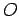on the optic axis which lies midway between the two bounding surfaces is called the optic centre.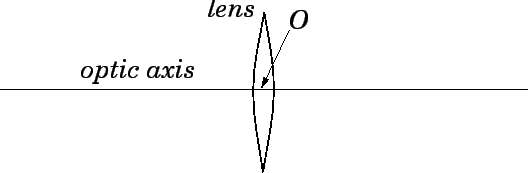There are two basic kinds of lenses: converging, and diverging. A converging lens brings all incident light-rays parallel to its optic axis together at a point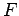, behind the lens, called the focal point, or focus, of the lens. A diverging lens spreads out all incident light-rays parallel to its optic axis so that they appear to diverge from a virtual focal pointin front of the lens. Here, the front side of the lens is conventionally defined to be the side from which the light is incident. The differing effects of a converging and a diverging lens on incident light-rays parallel to the optic axis (i.e., emanating from a distant object) are illustrated in Fig. 77.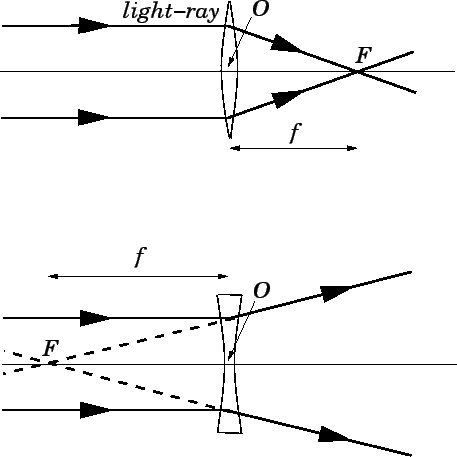Lenses, like mirrors, suffer from spherical aberration, which causes light-rays parallel to the optic axis, but a relatively long way from the axis, to be brought to a focus, or a virtual focus, closer to the lens than light-rays which are relatively close to the axis. It turns out that spherical aberration in lenses can be completely cured by using lenses whose bounding surfaces are non-spherical. However, such lenses are more difficult, and, therefore, more expensive, to manufacture than conventional lenses whose bounding surfaces are spherical. Thus, the former sort of lens is only employed in situations where the spherical aberration of a conventional lens would be a serious problem. The usual method of curing spherical aberration is to use combinations of conventional lenses (i.e., compound lenses). In the following, we shall make use of the paraxial approximation, in which spherical aberration is completely ignored, and all light-rays parallel to the optic axis are assumed to be brought to a focus, or a virtual focus, at the same point. This approximation is valid as long as the radius of the lens is small compared to the object distance and the image distance.

The focal length of a lens, which is usually denoted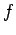, is defined as the distance between the optic centreand the focal point, as shown in Fig. 77. However, by convention, converging lenses have positive focal lengths, and diverging lenses have negative focal lengths. In other words, if the focal point lies behind the lens then the focal length is positive, and if the focal point lies in front of the lens then the focal length is negative.

Consider a conventional lens whose bounding surfaces are spherical. Let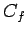be the centre of curvature of the front surface, and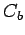the centre of curvature of the back surface. The radius of curvature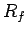of the front surface is the distance between the optic centreand the point. Likewise, the radius of curvature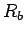of the back surface is the distance between pointsand. However, by convention, the radius of curvature of a bounding surface is positive if its centre of curvature lies behind the lens, and negative if its centre of curvature lies in front of the lens. Thus, in Fig. 78,is positive andis negative.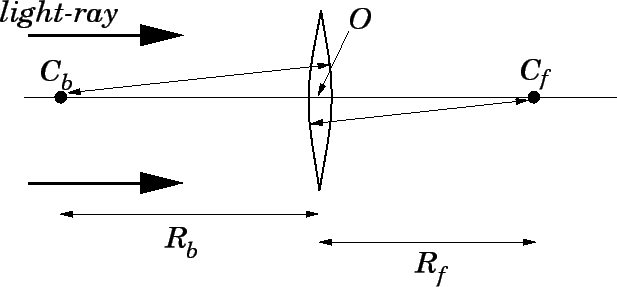In the paraxial approximation, it is possible to find a simple formula relating the focal lengthof a lens to the radii of curvature,and, of its front and back bounding surfaces. This formula is written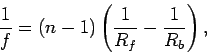(362)

whereis the refractive index of the lens. The above formula is usually called the lens-maker's formula, and was discovered by Descartes. Note that the lens-maker's formula is only valid for a thin lens whose thickness is small compared to its focal length. What Eq. (362) is basically telling us is that light-rays which pass from air to glass through a convex surface are focused, whereas light-rays which pass from air to glass through a concave surface are defocused. Furthermore, since light-rays are reversible, it follows that rays which pass from glass to air through a convex surface are defocused, whereas rays which pass from air to glass through a concave surface are focused. Note that the net focusing or defocusing action of a lens is due to the difference in the radii of curvature of its two bounding surfaces.

Suppose that a certain lens has a focal length. What happens to the focal length if we turn the lens around, so that its front bounding surface becomes its back bounding surface, and vice versa? It is easily seen that when the lens is turned around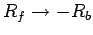and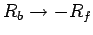. However, the focal lengthof the lens is invariant under this transformation, according to Eq. (362). Thus, the focal length of a lens is the same for light incident from either side. In particular, a converging lens remains a converging lens when it is turned around, and likewise for a diverging lens.

The most commonly occurring type of converging lens is a bi-convex, or double-convex, lens, for which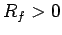and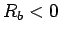. In this type of lens, both bounding surfaces have a focusing effect on light-rays passing through the lens. Another fairly common type of converging lens is a plano-convex lens, for whichand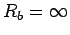. In this type of lens, only the curved bounding surface has a focusing effect on light-rays. The plane surface has no focusing or defocusing effect. A less common type of converging lens is a convex-meniscus lens, for whichand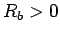, with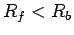. In this type of lens, the front bounding surface has a focusing effect on light-rays, whereas the back bounding surface has a defocusing effect, but the focusing effect of the front surface wins out.

The most commonly occurring type of diverging lens is a bi-concave, or double-concave, lens, for which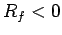and. In this type of lens, both bounding surfaces have a defocusing effect on light-rays passing through the lens. Another fairly common type of converging lens is a plano-concave lens, for whichand. In this type of lens, only the curved bounding surface has a defocusing effect on light-rays. The plane surface has no focusing or defocusing effect. A less common type of converging lens is a concave-meniscus lens, for whichand, with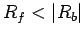. In this type of lens, the front bounding surface has a defocusing effect on light-rays, whereas the back bounding surface has a focusing effect, but the defocusing effect of the front surface wins out.

Figure 79 shows the various types of lenses mentioned above. Note that, as a general rule, converging lenses are thicker at the centre than at the edges, whereas diverging lenses are thicker at the edges than at the centre.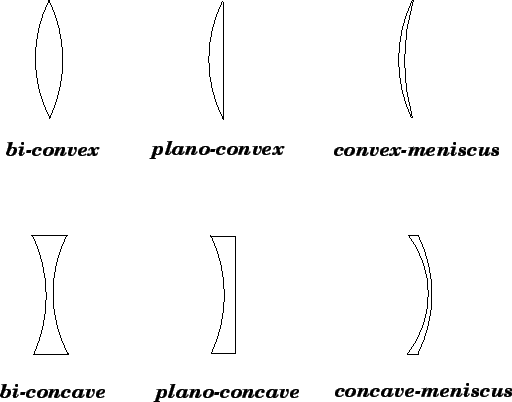Next: Image Formation by Thin Up: Paraxial Optics Previous: Image Formation by Plane
Richard Fitzpatrick 2007-07-14